Documentation

## Adaptive Noise Cancellation Using ANFIS

This example shows how to do adaptive nonlinear noise cancellation using the `anfis` and `genfis` commands.

### Signal and Noise

Define a hypothetical information signal, `x`, sampled at 100Hz over 6 seconds.

```time = (0:0.01:6)'; x = sin(40./(time+0.01)); plot(time,x) title('Information Signal x','fontsize',10) xlabel('time','fontsize',10) ylabel('x','fontsize',10)```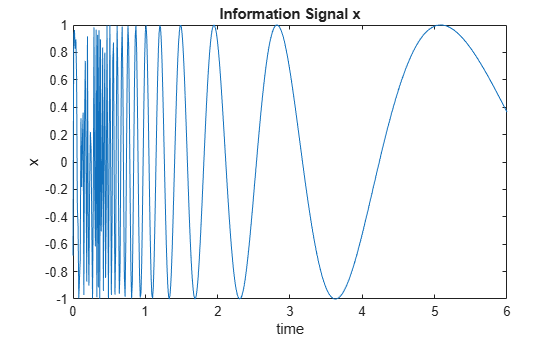Assume that `x` cannot be measured without an interference signal, ${\mathit{n}}_{2}$, which is generated from another noise source, ${\mathit{n}}_{1}$, via a certain unknown nonlinear process.

The plot below shows noise source ${\mathit{n}}_{1}$.

```n1 = randn(size(time)); plot(time,n1) title('Noise Source n_1','fontsize',10) xlabel('time','fontsize',10) ylabel('n_1','fontsize',10)```Assume that the interference signal, ${\mathit{n}}_{2}$, that appears in the measured signal is generated via an unknown nonlinear equation:

`${n}_{2}\left(k\right)=\frac{4\mathrm{sin}\left({n}_{1}\left(k\right)\right)\cdot {n}_{1}\left(k-1\right)}{1+{n}_{1}{\left(k-1\right)}^{2}}$`

Plot this nonlinear characteristic as a surface.

```domain = linspace(min(n1),max(n1),20); [xx,yy] = meshgrid(domain,domain); zz = 4*sin(xx).*yy./(1+yy.^2); surf(xx,yy,zz); xlabel('n_1(k)','fontsize',10); ylabel('n_1(k-1)','fontsize',10); zlabel('n_2(k)','fontsize',10); title('Unknown Interference Channel Characteristics','fontsize',10);```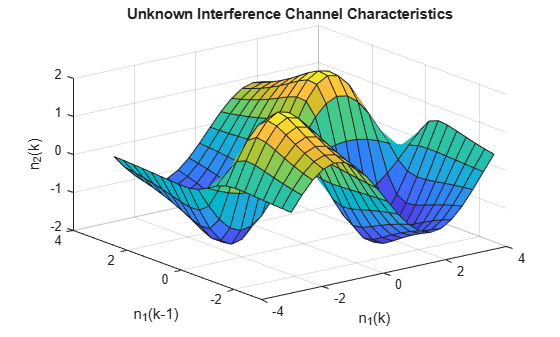Compute the interference signal, ${\mathit{n}}_{2}$, from the noise source, ${\mathit{n}}_{1}$, and plot both signals.

```n1d0 = n1; % n1 with delay 0 n1d1 = [0; n1d0(1:length(n1d0)-1)]; % n1 with delay 1 n2 = 4*sin(n1d0).*n1d1./(1+n1d1.^2); % interference subplot(2,1,1) plot(time,n1); ylabel('noise n_1','fontsize',10); subplot(2,1,2) plot(time,n2); ylabel('interference n_2','fontsize',10);```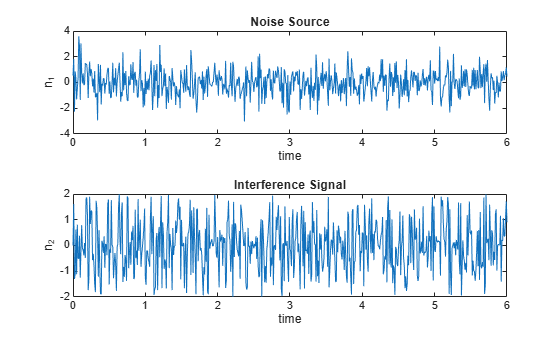${\mathit{n}}_{2}$ is related to ${\mathit{n}}_{1}$ via the highly nonlinear process shown previously; from the plots, it is hard to see if these two signals are correlated in any way.

The measured signal, `m`, is the sum of the original information signal, `x`, and the interference, ${\mathit{n}}_{2}$. However, we do not know ${\mathit{n}}_{2}$. The only signals available to us are the noise signal, ${\mathit{n}}_{1}$, and the measured signal `m`.

```m = x + n2; % measured signal subplot(1,1,1) plot(time, m) title('Measured Signal','fontsize',10) xlabel('time','fontsize',10) ylabel('m','fontsize',10)```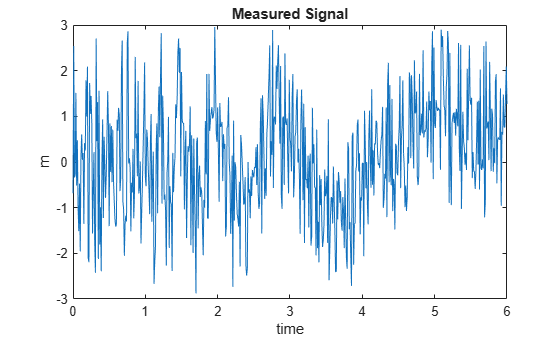You can recover the original information signal, `x`, using adaptive noise cancellation via ANFIS training.

### Build the ANFIS Model

Use the `anfis` command to identify the nonlinear relationship between ${\mathit{n}}_{1}$ and ${\mathit{n}}_{2}$. While ${\mathit{n}}_{2}$ is not directly available, you can assume that `m` is a "contaminated" version of ${\mathit{n}}_{2}$ for training. This assumption treats `x` as "noise" in this kind of nonlinear fitting.

Assume the order of the nonlinear channel is known (in this case, `2`), so you can use a 2-input ANFIS model for training.

Define the training data. The first two columns of `data` are the inputs to the ANFIS model, ${\mathit{n}}_{1}$ and a delayed version of ${\mathit{n}}_{1}$. The final column of `data` is the measured signal, `m`.

```delayed_n1 = [0; n1(1:length(n1)-1)]; data = [delayed_n1 n1 m];```

Generate the initial FIS object. By default, the grid partitioning algorithm uses two membership functions for each input variable, which produces four fuzzy rules for learning.

```genOpt = genfisOptions('GridPartition'); inFIS = genfis(data(:,1:end-1),data(:,end),genOpt);```

Tune the FIS using the `anfis` command with an initial training step size of `0.2`.

```trainOpt = anfisOptions('InitialFIS',inFIS,'InitialStepSize',0.2); outFIS = anfis(data,trainOpt);```
```ANFIS info: Number of nodes: 21 Number of linear parameters: 12 Number of nonlinear parameters: 12 Total number of parameters: 24 Number of training data pairs: 601 Number of checking data pairs: 0 Number of fuzzy rules: 4 Start training ANFIS ... 1 0.761817 2 0.748426 3 0.739315 4 0.733993 5 0.729492 Step size increases to 0.220000 after epoch 5. 6 0.725382 7 0.721269 8 0.717621 9 0.714474 Step size increases to 0.242000 after epoch 9. 10 0.71207 Designated epoch number reached --> ANFIS training completed at epoch 10. Minimal training RMSE = 0.712070 ```

The tuned FIS, `outFIS`, models the second-order relationship between ${\mathit{n}}_{1}$ and ${\mathit{n}}_{2}$.

### Evaluate Model

Calculate the estimated interference signal, `estimated_n2`, by evaluating the tuned FIS using the original training data.

`estimated_n2 = evalfis(outFIS,data(:,1:2));`

Plot the and actual ${\mathit{n}}_{2}$ signal and the estimated version from the ANFIS output.

```subplot(2,1,1) plot(time, n2) ylabel('n_2 (unknown)'); subplot(2,1,2) plot(time, estimated_n2) ylabel('Estimated n_2');```The estimated information signal is equal to the difference between the measured signal, `m`, and the estimated interference (ANFIS output).

`estimated_x = m - estimated_n2;`

Compare the original information signal, `x`, and the estimate, `estimated_x`.

```figure plot(time,estimated_x,'b',time,x,'r') legend('Estimated x','Actual x (unknown)','Location','SouthEast')```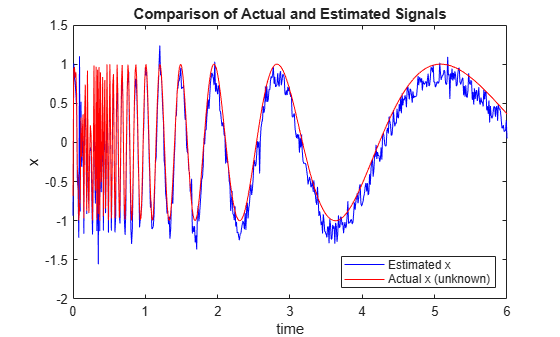Without extensive training, the ANFIS produces a good estimate of the information signal.